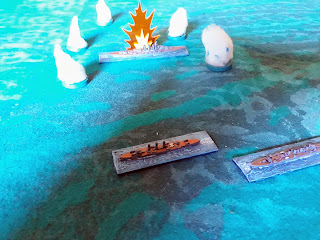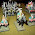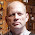## Wednesday, 17 July 2019

### 1 hit for 10 damage, or 10 hits for 1 damage each?

Many wargames have a mechanic that requires a number of damage points to be inflicted for a target to be eliminated. Damage is usually caused by a number of shots, each shot having a certain probability of hitting the target, and each shot causing a number of damage points. Thus, we already have various variables with which we can shape our mechanic: the number of total damage points needed to kill the target; the probability of each shot causing a hit; and the number of damage points per successful hit. Given these variables, it is quite naturally to ask how long it will take before the target is eliminated. And is it better to have a mechanic in which we need 10 hits, causing 1 damage each, or needing one mega-hit causing 10 damage by iself?2 cruisers closing in on a battleship ...Do we prefer many shots, many of them on target, but each doing a tiny amount of damage?... or do we rather prefer many shots missing, but the one shot that hits, causing the ship to sink?
Mathematics

To get a good insight in the mechanic, let's take a look at the math. First, let us define some of the parameters:
• p_success: the probability (a number between 0 and 1) with which a shot hits the target. Let's assume p_success is the same for all shots.Translated into a die roll, a D6 that requires 5+ to hit has p_success = 0.33, a D10 that requires 4+ to hit sets p_success equal to 0.6, and so on.
• s: the number of successful shots needed to kill the target. Again, to keep things simple, let's assume that all hits cause the same amount of damage d. Thus, if the target has D damage points, s = D/d (rounded up). E.g., if a successful shot causes 3 damage, and we shoot at a 10-damage target, we need 4 successful shots (10/3, rounded up) to kill the target.
• n: the total number of shots (trials), included misses. Not every shot will hit the target, as is obvious from p_success. n will always greater or equal than s. If we are lucky, we can kill the target with s number of shots, but the lower the value of p_success, the more shots we will need to reach s successful shots.
So, we can phrase the mathematical problem as follows: what's the probability of s hits occurring in a sequence of n shots, with the last shot being successful (and thus killing the target)? Or to put it differently: what is the probability we will need n shots, given s successful shots with the n-th shot being the last successful s-th shot?

This problem might look familiar to another problem we have analyzed before, the Buckets of Dice procedure. When rolling  n dice, we are often interested in how many dice will score a success given a certain probability of success for each dice. The binomial distribution expresses the probability that we score s successes, given n dice. But now, we want something slightly different. Instead of fixing the number of trials n, and keeping the number of successes as a changing variable, we now want to fix the required number of successes s, and consider the total number of trials n as a variable. It is a minor change of viewpoint, but we can still use the same mathematical framework.

Before we can write down the equations, we need some additional insights:
• The first insight we need is that it doesn't matter which of our shots are successful, except for the last one. If we want to reach 4 successful hits in 10 trials, we know that the 10th shot needs to be a success (the 4th hit), but the previous 3 hits can happen anywhere in the previous sequence of 9 shots.
• The second insight is that if 3 shots can happen anywhere in a sequence of 9 shots, the mathematics don't care whether those shots are taken in sequence or all together, as long as all shots are independent from each other. Thus, we can use the binomial distribution as described by the Buckets of Dice method to describe the probability distribution of s-1 shots being succcessful, out of n-1 total number of shots. Using the same notation as in our Buckets of Dice blogpost, we can write this distribution as Bin(s-1, n-1, p_success).
Now we need to combine both observations. The first s-1 succesful hits can happen anywhere within the first n-1 shots. The n-th shot must be the s-th succesful hit, and this shot will be succesful with a probability equal to p_success. Thus, the complete probability distribution for s succesful hits, using n shots, with the last being a success, can be written as:

NBin(s, n , p_success) = Bin(s-1, n-1, p_success) * p_success

Such a distribution is known in mathematics as a Negative Binomial Distribution, hence the notation Nbin (see also the appendix for some more information).

The relationship with the more well-known Binomial Distribution is clear, but as mentioned before, the viewpoint is slightly different. The Binomial Distribution is mostly concerned with the probability of scoring k successes out of n trials; the Negative Binomial distribition is interested in the probability of needing n trials to score s successes.

Analysis

So, what does this distribution look like? Many spreadsheet programs have the Negative Binomial Distribution built in, but you can also use the above formulation, expressing it as the product of the Binomial Distribution (which also often is a pre-defined function in many spreadsheet programs) multiplied by the probability p_success.

Let's look at some simple cases first, just to get a feeling of how this function evolves with various parameter settings.

Let's first set p_success = 2/6, which means rolling 5 or 6 on a D6 in order to score a hit. Let us further assume we want to score 2 successful hits. The graph below shows the probability of number of total shots n needed.
As you can see, there is zero probability n equals 1 (obviously, since we need 2 successes), and n = 3 or n = 4 is the most likely outcome, each with roughly 15% chance of occuring. The probability we will need more than 4 trials decreases gradually.

Let us now assume we set p_success = 1/6, in other words, a successful hit will on average only happen once every 6 shots. The resulting distribution for the total number of shots n needed is shown below.
You can immediately see the most likely outcomes are n = 5 to 8, and again there is a decreasing probability we will need ever more shots, although the decrease is not as sudden as with p_success = 2/6.

Now let's very the number of required successful shots, while keeping p_success at 2/6. The graph below shows the probability distribution.
Since we need a higher number of successes, the graph shifts to right, in this case with a most likely outcome for n = 9 or 10, again with a gentle decrease for higher numbers of n.

I guess you are curious for other values of p_success and s as well, so here are the complete graphs. First, the probabilty disctributions for different values of p_success, fixing the number of successes on 2.
And here a variable number of successes, with fixing the probability for scoring a succesful hit:
Note the atypical shape of the distribution for 1 success, which is to be expected, since exactly the last shot needs to be a success, and all previous shots need to be misses.

Expected value for n

The above graphs might give you some insight in the mathematics, but how can we translate this into useful gaming mechanics? The first thing a game designer might be interested in, is the expected value of the total number of shots n (successes and misses), in function of p_success and s.

We will not go through the mathematical derivation, but the expected value for n (the expected value E(n) is the average value for n  if we would conduct or procedure an infinite number of times), equals s / p_success.

This is not so surprising, if we fill out some numbers:
• If p_success = 1/6, and s = 1 (we need one successful shot, we a chance of 1/6 of scoring one), we can expect to need 6 shots.
• If p_success = 3/6, and s = 2, then E(n) = 2*6/3 = 4, which means we can expect to need 4 shots in order to score to 2 successful hits with a success ratio of 50%.
So, what does this formula teach us?
• doubling the value of s, while keeping p_success a constant, will double the value of E(n). Or more generally, increasing or decreasing the value of s by a certain ratio, will also multiply E(n) by that same ratio.
• doubling the value of p_success, while keeping s a constant, will halve the value of E(n). Or more generally, increasing or decreasing p_success by a certain ratio, will inversely proportional change the value of E(n).
If we know put some more gaming terms into our equation, and if we want to keep the initial example of having to score a number of hits D on a target, with each hit inflicting d damage points, we can say that s = D/d. But since E(n) = s / p_success, we can now say that:

E(n) = D / (p_success * d)

Thus, we have the expected number of shots (which can translate in a number of turns in the game), the total amount of damage needed, the chance for scoring a hit, and the damage per hit, in one nice formula. You can play around with the values, keeping some fixed while changing others, and see what the outcome is.

Standard deviation
The standard deviation of a stochastic process is a measure for how far any given experiment can deviate from the expected value. After all, the expected value is only an average number, but any single experiment can produce a number lower or higher than the expected value.

The standard deviation for n, is given by the square root of (1 - p_success) * s / (p_success * p_success). This seems like a rather convoluted formula, and you might want to plug in some numbers to see how the standard deviation will change with various parameters, but you can see that the standard deviation:
• ... increases proportional to the square root of s  if p_success is kept constant. Thus, quadrupling s, will only double the standard deviation.
• ... decreases with higher values of p_success, due to p_success in the denominator. Roughly we can say that the standard deviation changes inversely proportional to p_success.
A full analysis would lead us to far, but again you can try out some numbers yourself. One last thing to note is that you can also compute the relative value of the standard deviation vs E(n). This turns out to sqrt ((1-p_success)/s). Thus, increasing the number or s, while keeping p_success a constant, will make the relative spread narrower around E(n).

Gaming mechanics

What does this all mean for gaming mechanics?

We know that E(n) = D / (p_success * d). Now suppose we want to find values for the different parameters, but keeping the expected value E(n), which can act as a proxy for the number of turns needed to sink a target, the same. Also suppose we keep D a constant (after all, D the total number of damage points, and is in some sense an arbitrary number). For 2 different gaming mechanics, each with different values for d and p_success, and forcing E(n) to remain a constant, we can then say:

p_success_1 * d_1 = p_success_2 * d_2

Thus, the damage points per successful shot should scale inversely proportional to the probability of a successful shot. If we set p_success_1 = 2/6, and d_1 = 6 points, then this is equivalent to setting p_success = 4/6, and d_2 = 3 points.

When we say both procedures are equivalent, they are both equivalent in E(n), the expected number of shots needed to sink the target. But there will be a difference in the standard deviation. However, to compute the standard deviation, we need to set a value for s. s is determined by D and d, since s = D/d. So, the standard deviation, equalling sqrt((1-p_success)*s/p_success*p_success) is now rewritten as sqrt((1-p_success)*D/p_success*p_success*d).

So, let's plug in some numbers, and let's set D at 12:
• p_success = 1/6, d = 12 => s = 1, E(n) = 6, stdev = 5.47
• p_success = 2/6, d = 6 => s = 2, E(n) = 6, stdev = 3.46
• p_success = 4/6, d = 3 => s = 4, E(n) = 6, stdev = 1.73
The graph for these 3 settings is shown below.

Playing around with the numbers is of course fun, but there are others things to consider. E.g. setting d = D (i.e. a single shot kills the target), means avoiding bookkeeping (tracking the number of hits, damage points left, ...). Setting d different from D implies keeping track of the amount of damage inflicted. Whether or not that's a good thing, depends on other mechanisms in the rules.

Conclusion

Whether you want one big shoot that kills in an instant, or a sequence of low intensity shots that require many turns to kill, keep in mind that what really matters is the expected number of shots (misses and hits) needed, as well as the standard deviation on that number.

Appendix:
1. The Negative Binomial Distribution is described in various ways in different textbooks. Often, it is defined as a fixed number of failures in a sequence of trials, thereby reversing the definition of success and failure as we have used in this blogpost. Sometimes, instead of the total number trials n, the number of failures and number of successes are used as parameters, wit n being the sum of the two. But it all ends up describing the same type of distribution. For more information, see https://en.wikipedia.org/wiki/Negative_binomial_distribution

1.1.